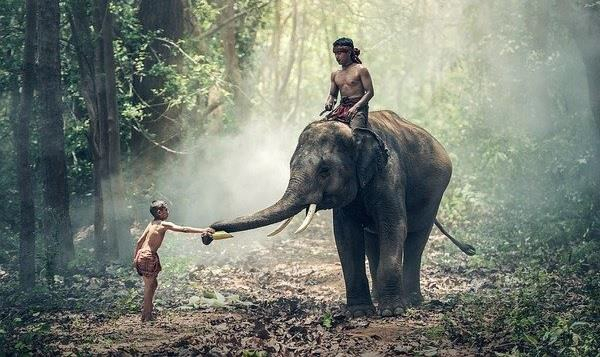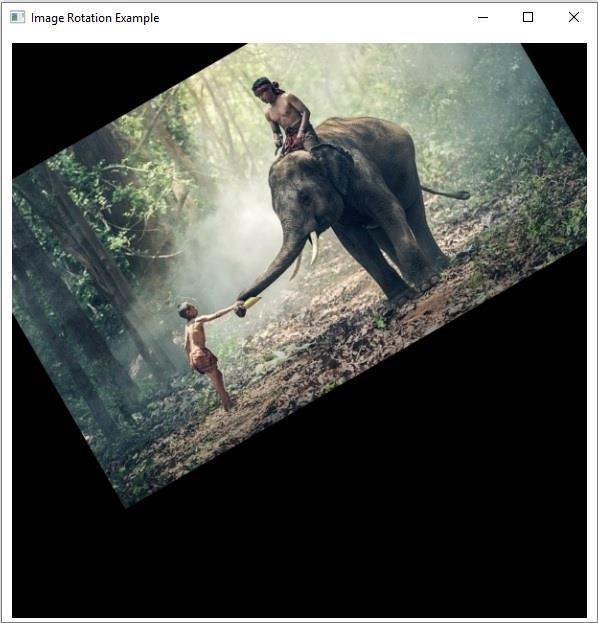# How to rotate an image with OpenCV using Java?

The warpAffine() method of the Imgproc class applies an affine transformation to the specified image. This method accepts −

• Three Mat objects representing the source, destination, and transformation matrices.

• An integer value representing the size of the output image.

To rotate an image Create a rotation matrix and pass it as a transformation matrix to this method along with the other parameters.

## Example

import java.awt.Image;
import java.awt.image.BufferedImage;
import java.io.IOException;
import javafx.application.Application;
import javafx.embed.swing.SwingFXUtils;
import javafx.scene.Group;
import javafx.scene.Scene;
import javafx.scene.image.ImageView;
import javafx.scene.image.WritableImage;
import javafx.stage.Stage;
import org.opencv.core.Core;
import org.opencv.core.Mat;
import org.opencv.core.Point;
import org.opencv.core.Size;
import org.opencv.highgui.HighGui;
import org.opencv.imgcodecs.Imgcodecs;
import org.opencv.imgproc.Imgproc;
public class RotatingAnImage extends Application {
public void start(Stage stage) throws IOException {
String file ="D:\Images\elephant.jpg";
//Creating destination matrix
Mat dst = new Mat(src.rows(), src.cols(), src.type());
// Creating a Point object
Point point = new Point(300, 200);
//Creating the transformation matrix
Mat rotationMatrix = Imgproc.getRotationMatrix2D(point, 30, 1);
// Creating the object of the class Size
Size size = new Size(src.cols(), src.cols());
// Rotating the given image
Imgproc.warpAffine(src, dst, rotationMatrix, size);
//Converting matrix to JavaFX writable image
Image img = HighGui.toBufferedImage(dst);
WritableImage writableImage= SwingFXUtils.toFXImage((BufferedImage) img, null);
//Setting the image view
ImageView imageView = new ImageView(writableImage);
imageView.setX(10);
imageView.setY(10);
imageView.setFitWidth(575);
imageView.setPreserveRatio(true);
//Setting the Scene object
Group root = new Group(imageView);
Scene scene = new Scene(root, 595, 400);
stage.setTitle("Image Rotation Example");
stage.setScene(scene);
stage.show();
}
public static void main(String args[]) {
launch(args);
}
}

## Input Image## Output

On executing the above program, it generates the following output −